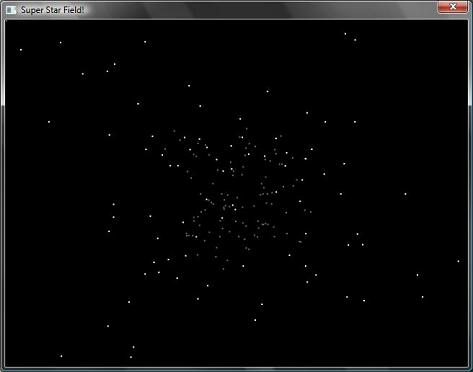Sample of the Week, II

This week's featured sample comes from Jeff Sidlosky. It is a star field simulation - you know the one where it appears like you're moving fast through space, with stars whizzing past you.

```' Star-field simulation
Initialize()
Main()
Sub Initialize
GraphicsWindow.Title = "Super Star Field!"
GraphicsWindow.Width = 640
GraphicsWindow.Height = 480
GraphicsWindow.BackgroundColor = "black"
GraphicsWindow.PenWidth = 0
GraphicsWindow.Show()
num_stars = 200
For index = 0 To num_stars
NewStar()
EndFor
EndSub
Sub NewStar
Array.SetValue("star_x", index, Math.GetRandomNumber(100) - 50)
Array.SetValue("star_y", index, Math.GetRandomNumber(100) - 50)
' Pick a random z depth
z = (Math.GetRandomNumber(50) / 100) + 0.50
Array.SetValue("star_z", index, z)
GraphicsWindow.BrushColor = "DimGray"
Array.SetValue("star_color", index, 0)
EndSub
Sub Update
For index = 0 To num_stars
z = Array.GetValue("star_z", index)
x = (Array.GetValue("star_x", index) / z) + 320
y = (Array.GetValue("star_y", index) / z) + 240
shape = Array.GetValue("star_shape", index)
' Next z position
z = z - 0.02
If(x < 0 Or x > 639 Or y < 0 Or y > 479 Or z <= 0) Then
GraphicsWindow.RemoveShape(shape)
NewStar()
Else
' Check if we should make the star brighter
If(z < 0.4) Then
If(Array.GetValue("star_color", index) = 0) Then
GraphicsWindow.BrushColor = "White"
GraphicsWindow.RemoveShape(shape)
Array.SetValue("star_shape", index, shape)
Array.SetValue("star_color", index, 1)
EndIf
EndIf
GraphicsWindow.MoveShape(shape, x, y)
Array.SetValue("star_z", index, z)
EndIf
EndFor
EndSub
Sub Main
' Run forever
While(1 = 1)
Update()
EndWhile
EndSub
```

And here's the screenshot! The picture really doesn't do justice. Try this program on your copy of Small Basic to experience the real deal.Do you want your samples to be featured here? Post them in our forums and we'll pick one each week.

Tags

1.anonymouscommenter says:
2.anonymouscommenter says:

I’m having this error when I try to use the command line compiler:

The process cannot access the file ‘.SmallBasicLibrary.dll’ because it is being used by another process.

I think the problem is in CopyLibraryAssemblies, because in this case, the method is trying to copy a Assembly that already in use into the same destination folder.

3.anonymouscommenter says:

Please note that if you try this sample code in the latest Small Basic version, you will need to refactor it a little, since some of the methods previously contained in the GraphicsWindow class have been moved (and sometimes renamed) in the Shapes class.

4.anonymouscommenter says:

Just tried the programe out, doesn't seem to work?

5.Uberlan_GS says:

Try this…

' Star-field simulation

Initialize()

Main()

Sub Initialize

GraphicsWindow.Title = "Super Star Field!"

GraphicsWindow.Width = 640

GraphicsWindow.Height = 480

GraphicsWindow.BackgroundColor = "black"

GraphicsWindow.PenWidth = 0

GraphicsWindow.Show()

num_stars = 200

For index = 0 To num_stars

NewStar()

EndFor

EndSub

Sub NewStar

Array.SetValue("star_x", index, Math.GetRandomNumber(100) – 50)

Array.SetValue("star_y", index, Math.GetRandomNumber(100) – 50)

' Pick a random z depth

z = (Math.GetRandomNumber(50) / 100) + 0.50

Array.SetValue("star_z", index, z)

GraphicsWindow.BrushColor = "DimGray"

Array.SetValue("star_color", index, 0)

EndSub

Sub Update

For index = 0 To num_stars

z = Array.GetValue("star_z", index)

x = (Array.GetValue("star_x", index) / z) + 320

y = (Array.GetValue("star_y", index) / z) + 240

shape = Array.GetValue("star_shape", index)

' Next z position

z = z – 0.02

If(x < 0 Or x > 639 Or y < 0 Or y > 479 Or z <= 0) Then

Shapes.Remove(shape)

NewStar()

Else

' Check if we should make the star brighter

If(z < 0.4) Then

If(Array.GetValue("star_color", index) = 0) Then

GraphicsWindow.BrushColor = "White"

Shapes.Remove(shape)

Array.SetValue("star_shape", index, shape)

Array.SetValue("star_color", index, 1)

EndIf

EndIf

Shapes.Move(shape, x, y)

Array.SetValue("star_z", index, z)

EndIf

EndFor

EndSub

Sub Main

' Run forever

While(1 = 1)

Update()

EndWhile

EndSub